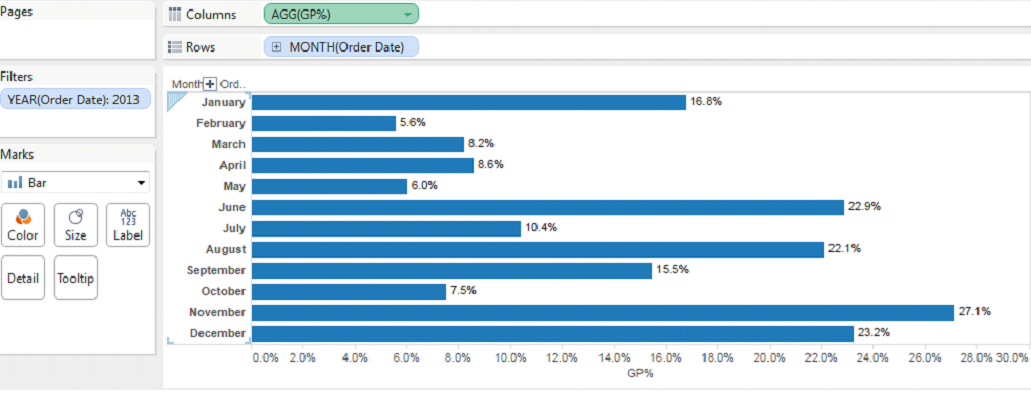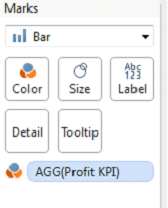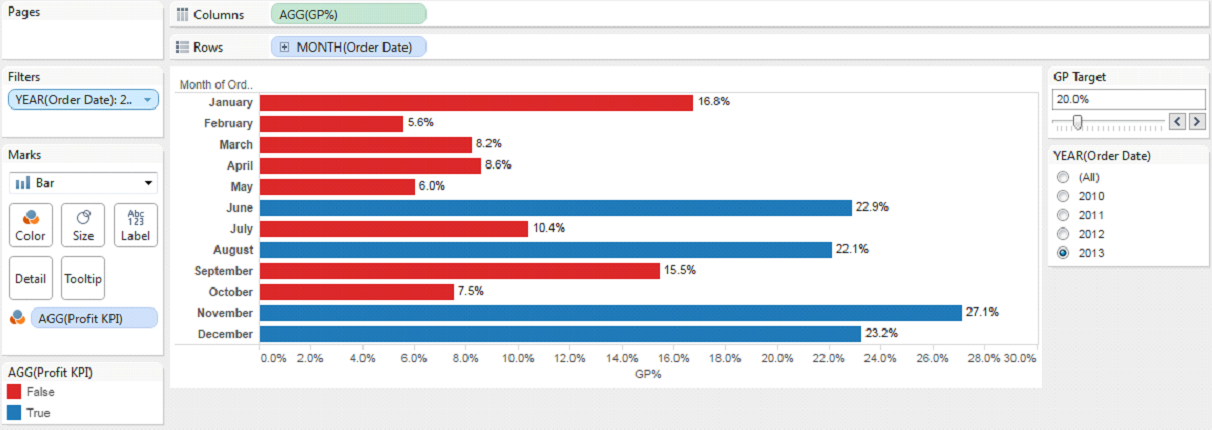# What If Analysis Scenario in Tableau

Parameters are one of the most powerful features available in Tableau to analyze and interact with data. Parameters are dynamic values that can replace constant values in calculations. For example, you may create a calculated field that returns true if Sales is greater than \$500,000 and otherwise return false. You can replace the constant value of “500,000” in the formula with a parameter that you can change dynamically using the parameter control.

In this post we will use parameters feature to create a “What-if” analysis. A “What-if” analysis is the process of changing the values a parameter to see how those changes will affect the outcome of data in a Tableau worksheet.

Let’s use superstore data that comes with Tableau desktop. We will analyze Gross Profit % (GP%) from the data set and build a KPI that would help us in identifying months that have exceeded the Profit Target set by a parameter.

• Create a calculated field with GP% = sum([Profit])/sum([Sales])
• Filter out the data for 2013 year in “Filters” pane. Also, setup a quick filter with single value list
• Create a view like below with order date in row shelf and GP% in column shelf•  To create a parameter, right click in the Parameters window in the bottom left corner of the sheet view and select “Create Parameter”. You will have six data type options to choose from. We will select float data type as GP% is best represented in decimals. Name the parameter as GP Target. Let’s keep the current value as 0 (which will be default value when you use parameter for the first time). Choose display format as “percentage” with one decimal point. Giving a “range” would specify the lower and upper limit of the parameter. Here we will take it as 0 and 1, where 1 being highest (100%) and 0 being the lowest (0%) with increment of 5% (0.05 step size).•  A parameter is a dependent variable which means it does not do anything on its own. There has to be a condition defined that would allow us to use the parameter. In this what-if analysis we want to see whether GP% was achieved in a particular month or not. So, we will create a calculated field that would satisfy the condition if the GP% is greater or equal to GP Target• Drag the calculated field “Profit KPI” from the above step and drop it in color shelf of marks card• Last step is to right click on the parameter “GP Target” and select “show parameter control”
Your first what-if analysis is ready! As you move the “GP Target” slider, you will have the months highlighted (in blue or red) if they have achieved the profit target or not.